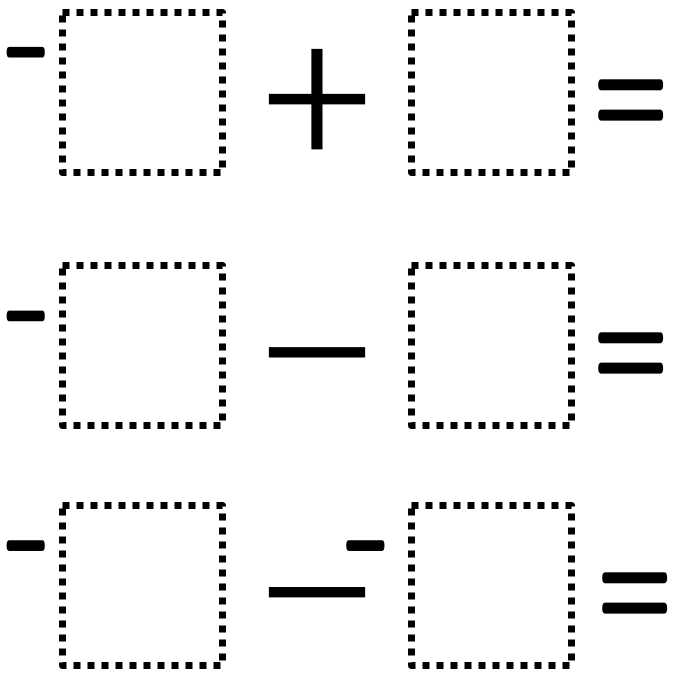Directions: Using the digits 1 to 6, at most one time each, fill in the boxes so that top two equations are equal and the bottom equation has the greatest value.### Hint

If you want the first two answers to be the same, should they both be positive or negative?
What pairs of numbers from 1-6 can you combine to make the same outcome?
How could we rewrite the second problem?
How could we rewrite the third problem?

First possible solution:
-6 + 3 = -3
-2 – 1 = -3 (2 and 1 in any order)
-4 – -5= 1 (or -5 – -4 = -1)

Second possible solution:
-6 + 1 = -5
-2 – 3 = -5 (2 and 3 in any order)
-4 – -5 = 1 (or -5 – -4 = -1)

Third possible solution:
-6 + 2 = -4
-1 – 3 = -4 (1 and 3 in any order)
-4 – -5 = 1 (or -5 – -4 = -1)

Source: Kate Nerdypoo

## Sides of a Triangle

Directions: The perimeter of a triangle is 20 units. Using whole numbers, how many sets …

1.Another one:

-6+2=-1-3=-4
and -4<-4-(-5)

•Great spot Dominique – I have added your suggestion to the list of solutions.

2.-6 + 5 = -1
-4 – (make it addition) 3 = -1
-2 – (make it addition) -1 (make positive) = -1
would also work

3.4.5.Sub 2 dogey63 on yt

6.-1 wouldn’t be the greatest possible value, would it?

7.Good questions, however I feel as though it would be better to refer to the three EXPRESSIONS, rather than equations.

So the directions would read like this “Using the digits 1 to 6, at most one time each, fill in the boxes so that top two expressions are equal and the bottom expression has the greatest value.

I’m not sure equations should be equal or greater than other equations, it is the expressions on either side of the equations which we compare.

8.Eliott Germain-Aubrey

1. -6+2=-4
2. -1-3=-4
3. -4= -4- -5